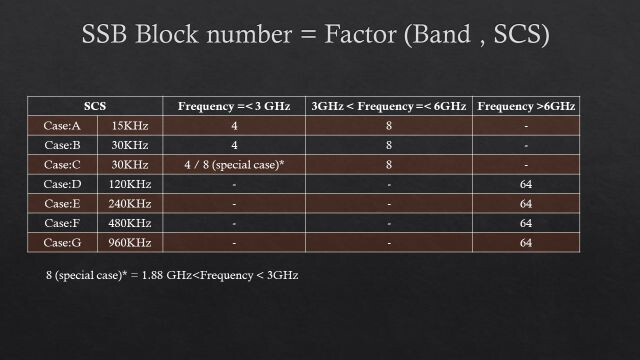# How many different beams are being transmitted in 5G-NR?

Actually to know how many beam can transmit in 5G NR , we need first to know how many SSB block can transmit within TDD frame and according we can know number of beams as equal to number of SSB block.

So, now the question is “how many SSB block can transmit within TDD frame?”. According to table 38.213 v17.2 3GPP, that mention clearly the number of SSB block is function of two factors: Band_Number & SSC (Subcarrier spacing).

Finally, let me consolidate below main conclusion points:

• One Time-slot in TDD frame 5G-NR carries Two SSB block.
• Every single beam needs at least one SSB block.
• Number of beams must be equal number of SSB blocks.

Reference:
38.213 v17.2 3GPP 5G | ShareTechnoteLinkedIn: